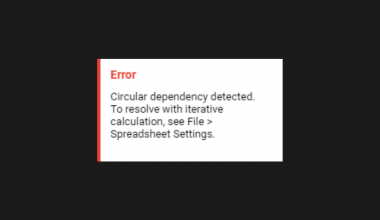The ADDRESS function in Google Sheets is useful if you want to return a cell’s address in the form of a text.

In other words, the function returns a cell reference or address as a text or string as per the specified row and column numbers.

## The Anatomy of the ADDRESS Function

The way we write the ADDRESS function is:

`=ADDRESS(row, column, [absolute_relative_mode], [use_a1_notation], [sheet]) `

• The equal sign `=`  is how we start any function in Google Sheets.
• `ADDRESS()` is our function. We need to add two attributes, namely the `row` and `column`, to make it work correctly. The other three attributes are optional, namely the `[absolute_relative_mode]`, `[use_a1-notation]`, and `[sheets]`.
• The `row` is the row number of the cell reference.
• The `column` is the column number of the cell reference. For example, “A” is column number “1”.
• The `[absolute_relative_mode]` is the indicator of whether the cell reference is row or column absolute. This attribute is optional.
• The  `[use_a1-notation]` is an expression used to indicate what style notation to display the cell reference in. This attribute is optional.
• The `[sheets]` is to indicate the name of the sheet of the cell reference. This attribute is optional.

Let’s have a more detailed understanding of what `[absolute_relative_mode]` and `[use_a1-notation]` mean.

#### Absolute Relative Mode:

This attribute is to determine whether the cell reference’s address would be relative or absolute.

Relative and absolute references behave differently when copied and filled to other cells.

Relative: changes when a formula is copied to another cell.

Absolute: remain constant, no matter where they are copied to.

Here are the references:This attribute is optional; hence it would be “1” by default.

#### Use A1 Notation:

This attribute is to determine how the cell reference’s address would be displayed.

Here are the references:#### Example 1:By using the `ADDRESS` function, the formula returns the cell address of “lemon” in the form of text.

As mentioned, without inputting the `[absolute_relative_mode]` attribute, it would be `1` by default, showing row and column absolute.

#### Example 2:By adding `false` as the `[use_a1_notation]` attribute, the cell reference address is displayed in the form of “R2C” instead of “A\$2”.

Besides, inputting `2` as the `[absolute_relative_mode]` attribute displays the row as absolute.

Do note that the value within the `[]` square bracket indicates that it is relative.

#### Example 3:By adding “Sheet1” into the formula, it will display which sheet the cell reference address is at.

## A Real Life Example of Using ADDRESS Function

Let’s use a real-life situation to utilize the `ADDRESS` function and combine all the components mentioned so far in practical use and show you how powerful this function can be!In this example, we use the `ADDRESS` function to determine which outlet has the minimum profit.

As shown, we incorporated `MATCH` and `MIN`  functions to evaluate which outlet needs further inspection or is no longer generating a profit.

1. Simply click on the cell that you want to write down your function at. In this example, it will be E3.1. Begin your function with an equal sign `=`, then followed by the name of the function, `ADDRESS`, then an open parenthesis `(`.1. We will then add the `MATCH` and `MIN` functions. The `MIN` function is to find the smallest numeric value in the range of profits from all outlets. The `MATCH` function would help match the smallest numeric value returned to the range of profits.1. We will then select D3 to D7, as this is the range of profits we will want to search the smallest numeric value from. The colon `:` is added to include all cells between the two endpoint cell references.1. Furthermore, we need to add a comma `,` to separate the `lookup_value` from our next attribute, `lookup_array`. We will then select D1 to D7, as this is the range of cells being searched to match the `lookup_value`.1. Next, add another comma `,` to separate the `lookup_value` from our next attribute, `match_type`In our case, we would like to find the first value that is exactly equal to the `lookup_value`. So we will add `0` for this expression.1. We then add a closing bracket `)` to close the `MATCH` function. Don’t forget to add a comma `,` to separate the `row` from `column`. To finish off the formula, we will add `4` as the column of cell reference is in Column D.Our final formula would look like this:

`=``ADDRESS(MATCH(MIN(D3:D7),D1:D7,0),4)`

1. After the following steps, your input should look like this:1. To give you a summary of how the formula was formed, here is a visual guide:You may make a copy of the spreadsheet using the link attached below and try it for yourself:

That’s about it! You can now use the ADDRESS function in Google Sheets together with the other numerous Google Sheets formulas to create even more powerful formulas that can make your life much easier.Our goal this year is to create lots of rich, bite-sized tutorials for Google Sheets users like you. If you liked this one, you'll love what we are working on! Readers receive ✨ early access ✨ to new content.

##### You May Also Like## How to Use IMAGINARY Function in Google Sheets

The IMAGINARY function in Google Sheets is a mathematical function that returns the imaginary coefficient of a given…

## How to Use IMPORTRANGE in Google Sheets

IMPORTRANGE is one of the most important functions in Google Sheets. It helps the user to handle and…## How to Fix ‘Circular Dependency Detected’ Error in Google Sheets

The circular dependency detected errors in Google Sheets is due to the formula being within the range you’re…## How to Convert Seconds to HH:MM:SS Format in Google Sheets

Converting seconds into a displayable HH:MM:SS format in Google Sheets can be hard, but once you learn how…